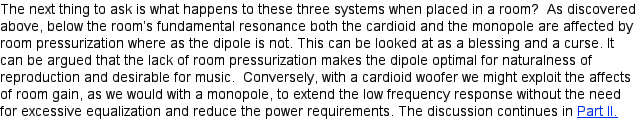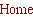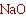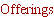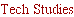Tech Design.....
___________________________

Room Response with Monopole, Dipole and Cardioid
Woofers, Part I: The Mechanics of Room Pressurization
.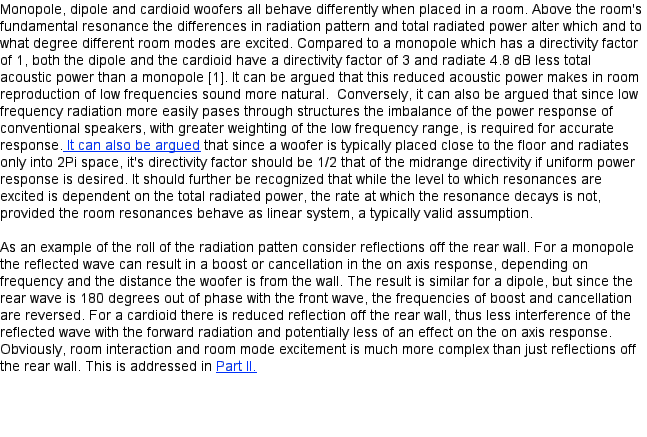The remaining factor is how the different  woofer systems interact with a room below the room’s
fundamental resonance. Below the room's fundamental resonance room pressurization becomes the
dominant means of reproducing low frequency. This is not to be confused with the increase in SPL
when a speaker is place close to a wall  or corner. It is well known that for a
sealed box woofer
system operating in
free space constant sound pressure vs. frequency requires that the driver
displacement increases as 1/f squared. That is, if the sound pressure level is to remain at a constant
level the driver displacement must increase by a factor of 4 for each octave the frequency is
decreased. This characteristic is satisfied by the natural behavior a typical driver operating above its
resonant frequency, in the mass controlled region, when a constant amplitude voltage is applied
across its terminals. However, below resonance the displacement asymptotes to a constant value vs.
frequency giving rise to the compliance controlled region of the response where a natural 2nd order
roll off of the sound pressure is observed. For dipoles and cardioids the additional cancellation
between front and rear sources results in a response that rolls off linearly with a 6dB/octave
decrease in output as the frequency drops. This requires an additional 6dB/octave equalization to
retain flat response in the mass controlled region with the result that the driver displacement must
increase as 1/f cubed. In the compliance controlled region the displacement must increase as 1/f to
retain the characteristic 2nd order acoustic roll off of a sealed box system.

When we bring these systems into a room, things change. For the sake of argument we shall assume
that the room is perfectly sealed and has perfectly rigid walls. We will ignore the effect of room modes
on the response for the moment. We are presently interested only in what happens to the sound
pressure when the frequency radiated by the woofer system is lower than the fundamental resonance
of the room, or when the wave length of the radiated sound is greater than twice the maximum room
dimension. Under such conditions the free field directivity index is meaningless. As the frequency
drops the pressure in the room varies in response to changes in the room volume. These changes,
though small, are the result of the motion of the driver's cone.

If we assume the temperature remains constant we can write,

PV = constant

where P is the pressure and V is the volume of the room. When a sealed box woofer system is in the
room the volume will vary as the driver’s cone moves in and out,

V = Vo - Sd X cos(ωt)

Here Vo is the volume of the room when the cone is at rest, Sd is the effective cone area and X is the
amplitude of the driver displacement.

From this, under the assumption that the pressure changes in the room are at constant temperature,
we can come to the result that

∆P ∙= -∆V ∙= ∆X

where ∆P, ∆V and ∆X are the changes in pressure, volume and driver displacement over a single
cycle. (The symbol, ∙=, is used to indicate “proportional to”.) What this tells us is that when the woofer
is operating below the room's fundamental resonance the pressure in the room is directly
proportional to the driver displacement. Thus, if the woofer system is chosen to have about the same
resonance as the room, rather than see a 2nd order roll off in the response below the woofer’s
resonance, in the compliance controlled region, room gain effects will augment the response and
tend to keep the sound pressure constant. This effect is well known behavior for sealed box woofer
systems and also accounts for exaggerate bass response if the resonance of the woofer system is
significantly below the room’s fundamental resonance. More on this later.

We can now ask ourselves, “How does room pressurization affect dipole and cardioid woofer
systems?” by examining how the woofer’s swept volume changes. First let’s look at a simple dipole
woofer system composed of two identical sources separated by some distance, d, much less than a
room dimension, and operating out of phase. In this case we can write

V = Vo – Sdf Xf cos(ωt) + Sdr Xr cos(ωt) = Vo

where the subscripts f and r indicate the front and rear source. Since Sd and X of both sources are
identical there is no net change in volume and,

∆P = -∆V ∙= ∆X = 0

Thus,
for a dipole operating below the room fundamental resonance there is no increase in
pressure
since the variation in the room volume over a cycle is zero. The ramifications are more
significant than they may first appear and will be discussed further in
Part II.

Now let’s examine the cardioid woofer system. Physically the cardioid woofer system is similar to the
dipole; two identical sources separated by a distance, d. But in addition to the separation the input
signal to the rear source is delayed by a time, td, such that

td = d/c

where c is the speed of sound. Thus we may write

V = Vo – Sdf Xf cos (ωt) + Sdr Xr cos(ω(t-td))

We can let

-ω td = Φ(ω)

which is just a frequency dependent phase angle and rewrite the equation for V as

V = Vo - Sd  X  (cos (ωt) - cos(ωt +Φ))

Through some trigonometric identities this can be expressed as

V = Vo - Sd  X  [cos (ωt)(1-cos(Φ)) + sin(Φ)sin(ωt )]

We still have the relationship

∆P = -∆V ∙= ∆X

but it is not clear what ∆X is. We do know that for a cardioid woofer the peak in the unequalized, on
axis frequency response occurs when Φ = π/2. This occurs at a frequency with wave length 4 times
longer than the separation between the sources, the quarter wave frequency. When Φ = π/2 the
equation above reduces to

V = Vo - √2 Sd X  sin(ωt + π/4)]

and

∆P = -∆V ∙=√2 ∆X

Thus the potential room gain effects are √2 greater than for a monopole woofer when operating at
the quarter wave frequency. However, Φ goes to zero as the frequency drops and, since cos(0) = 1
and sin(0) =0,  we find that there is no net volume change over a cycle; the same result as for a
dipole, as the frequency goes to zero. The question then arises as to the behavior of ∆X between the
quarter wave frequency and zero Hz. The question is relevant because, due to the nature of the of
the cardioid response above the quarter wave frequency the useful range of the cardioid woofer
system is limited to a maximum frequency of about ½ octave below the quarter wave frequency. (A
related limitation applies to a dipole.) Evaluation of the change in volume equation for the cardioid
yields the result shown in Figure 1 where the amplitude factor is the maximum value of the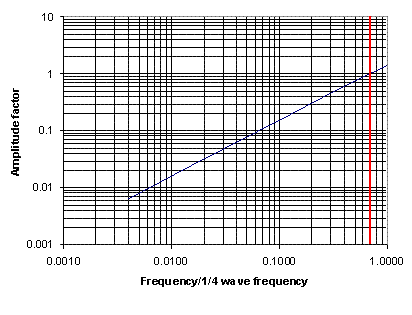Figure 1
[cos (ωt)(1-cos(Φ)) + sin(Φ)sin(ωt )] term over a cycle. The red line indicates the upper limit of the
useful range of the cardioid response. Figure 1 shows that ½ octave below the ¼ wave frequency the
potential room gain effect is identical to that of a monopole and as the frequency drops, the
displacement varies linearly with frequency. This means that at first glance it would appear that the
room gain effect would drop off at 6dB/octave compared to a monopole. Thus, for a monopole we see
a potential for room a room gain with an augmentation of 12dB/octave as the frequency decreases,
for cardioid the potential is an augmentation of 6dB/octave as the frequency drops and for a dipole
there is no room gain.

However, we must consider other factors. We must consider the behavior of the driver displacement
with frequency. We shall assume that the sources used to construct the monopole, dipole and
cardioid woofers all have the same free field response. As noted earlier, for a driver operating in the
mass controlled region above its resonance the displacement, X, varies as 1/ω squared. Below the
source’s resonance, in the compliance dominated region, the displacement asymptotes to a constant.
However, the phase relationships between the front and rear sources of dipole and cardioid woofer
systems leads to the need to apply additional equalization of 6dB/octave boost as the frequency
drops  to obtain the same free field response as the monopole. Thus, for the dipole and cardioid the
displacement will vary as 1/ω cubed in the mass controlled region and 1/ω in the compliance
controlled region. For the dipole this is of little consequence with regard to room pressurization since
regardless of how the excursion varies there is no net change in volume and no room pressurization
effect. On the other hand, for a cardioid we observe that when the frequency dependent driver
excursion is combined with the relationship given in Figure 1 for the multiplicative factor, the result is
that the volume equation reduces to the same relationship as that for a monopole. For a dipole, since
there is no change in volume there is not any response contribution due to room pressurization.
Thus
at first thought we might expect that the dipole response should follow the woofer's free
field response below the room's fundamental resonance.

The situation is different for a cardioid. First we must recognize that a cardioid is somewhat similar to
a dipole with regard to its free field response: 6dB/octave equalization is required. The result of this
equalization is also similar: the displacement varies as 1/ω cubed in the mass controlled region and
1/ω in the compliance dominated region. However, unlike a dipole the cardioid can be augmented by
room pressurization or room gain. As shown in Figure 1, the multiplicative factor due to the phase
variation from the time delay varies as ω.
The result is that the net behavior of the volume
displacement for an equalized cardioid is the same as a monopole.

The consequences of these behaviors are very interesting. Let us examine the response of
monopole, dipole and cardioid woofer systems which are designed to have the same on axis, free
filed response. For the sake of argument we can assume that these systems are composed of either
a single or dual monopole sources. The monopole is obviously a single source. The dipole will be
assumed to be composed of two sources separated by a distance, d, with the rear source 180
degrees out of phase with the front. The cardioid is identical to the dipole with the addition of a delay
of d/c added to the rear source. We shall further assume that the targeted on axis, anechoic
response is 2nd order, Q= 0.5, Fc = 20 Hz, and that the separation between the sources in the dipole
and cardioid is d = 18”. Figure 2 shows the response of the monopole (red), unequalized dipole
(green) and unequalized cardioid (blue). The first thing which is immediately evident is that the
unequalized dipole response is 6dB below that of the cardioid. This is a characteristic of a dipole and
cardioid of equal separation  and indicates that the such a dipole will require twice the driver
excursion of the cardioid to achieve the same on axis SPL and, assuming both use identical drivers,
6dB greater power. Figure 3 shows the equalized response of the dipole and cardioid along with the
required equalization to achieve the targeted on axis, free field response between 10 and 100 Hz.
The 90 dB line represents the 0 dB reference for the equalization. Aside form the observation that the
dipole equalization is uniformly 6dB greater that that for the cardioid, the equalization curves are
identical in shape. If we were to build the dipole system with twice the cone area or twice the driver
separation the difference in level would be negated. The important point is that relative to the 100 Hz
level, 14 dB of equalization is required at 20 Hz.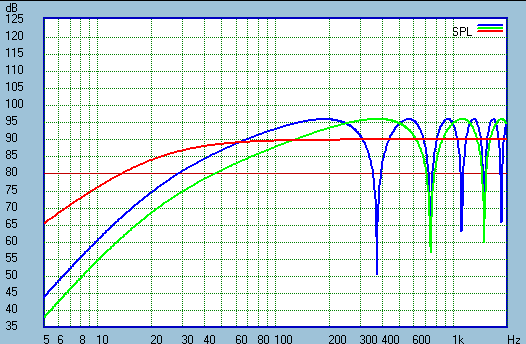Figure 2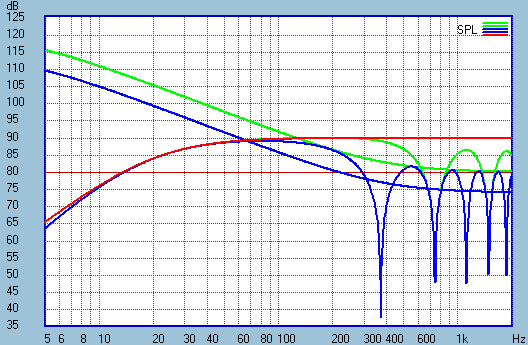Figure 3